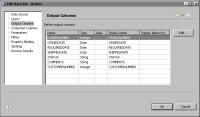Basic concepts
This section describes some of the basic concepts you need to understand and remember when writing JavaScript expressions. Understanding these concepts helps you avoid some common mistakes.
Data types
One of the fundamental concepts to understand is data types. Data types are the types of values—numbers, strings, and Booleans, for example—that can be represented and manipulated in any programming language. Every database field has a certain data type, every piece of report data has a certain data type, and every expression you create returns a value of a particular data type.
This concept is important because if an expression does not handle data types properly, errors occur or the report returns unexpected results. For example, you cannot perform mathematical calculations on numbers if they are of string type, and you cannot convert values in a date field to uppercase characters.
If writing an expression to manipulate a data set field, verify its type, particularly if the field values are numbers. Numbers can be of string or numeric type. For example, databases typically store zip codes and telephone numbers as strings. Item quantities or prices are always of numeric type so that the data can be manipulated mathematically. IDs, such as customer IDs or order IDs, are usually of numeric type so that the data can be sorted in numeric order, such as 1, 2, 3, 10, 11, rather than in alphanumeric order, such as 1, 10, 11, 2, 3.
To see the data type of a field, open the data set in Data Explorer, and choose Output Columns. Output Columns displays the fields in the data set and their types, as shown in Figure 11‑1.Figure 11‑1 Output Columns
Case sensitivity
JavaScript is a case-sensitive language. This feature means that a keyword, a function name, a variable name, or any other identifier must always be typed using the correct capitalization. For example, you must type the getDate( ) function as getDate( ), not as GetDate( ) or getdate( ). Similarly, myVar, MyVar, MYVAR, and myvar are four different variable names.
Data set field names are case-sensitive. When referring to a data set field in an expression, specify the field name with the same capitalization that the data source driver uses to identify the field. As mentioned previously, Output Columns in the data set editor shows the fields. If you use the expression builder to write an expression, select a field to insert in the expression to ensure that the correct field name is used.
Multiline expressions
An expression can contain multiple lines, as shown in the following example:
firstInitial = row["customerFirstname"].charAt(0);
firstInitial + ". " + row["customerLastname"];
The expression looks like lines of program code because it is. Expressions can be small pieces of code that do something. The expression in the previous example does the following tasks:It extracts the first character of a string value in a customerFirstname field and assigns the value to a variable named firstInitial.Then, it combines the firstInitial value, a period, a space, and the value in a customerLastname field.
An expression can contain as many lines as needed. Just remember that an expression returns a single value. If an expression contains several lines, it returns the results of the last line. The previous expression returns a value, such as T. Robinson.
The lines are called statements, and they are separated from each other by semicolons. If you place each statement on a separate line, as shown in the example, JavaScript allows you to leave out the semicolons. It is, however, good practice to use semicolons to separate statements.